### SUMIF Function In MS Excel

SUMIF function is used to sum cells based on one criteria. It can be used in many ways such as to sum
• Numbers
• Text
• Dates
Let's learn with Example

### How to Sum Numbers using SUMIF Function

>> SUMIF function below sums the values in the selected range that are less than selected Criteria.
For example, for sum values less than 40 , C7 equals =SUMIF(C2:C6,"<=40")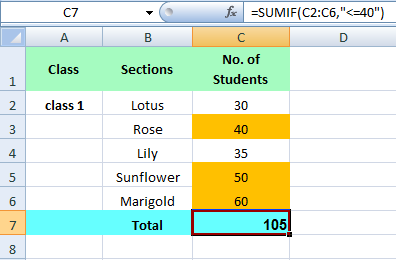>> The SUMIF function below sums values in the range if the corresponding cells in the other range contains the criteria value number.
For example, for sum values in the range D2:D7 if the corresponding cells in the range C2:C7 contain the value 50. E6 equals =SUMIF(C2:C7,50,D2:D7)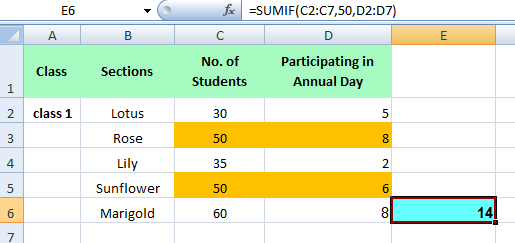### How to Sum Text using SUMIF Function

>> The SUMIF function below sums values in the range if the corresponding cells in the other range contains the criteria Text.
For example, for sum values in the range C2:C13 if the corresponding cells in the range B2:B13 contain the text Rose exactly . D13 equals =SUMIF(B2:B13,"Rose",C2:C13)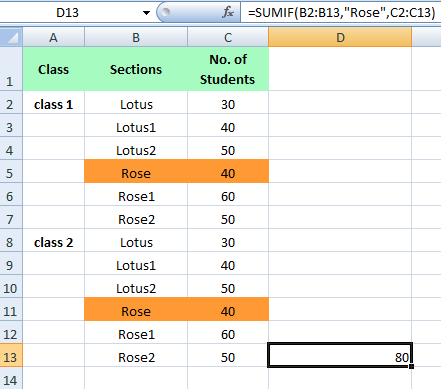>> The SUMIF function below sums values in the range if the corresponding cells in the other range contains the criteria Text +1 character.
For example, for sum values in the range C2:C13 if the corresponding cells in the range B2:B13 contain the text Rose +1 character . D13 equals =SUMIF(B2:B13,"Rose?",C2:C13)
A question mark (?) matches exactly one character.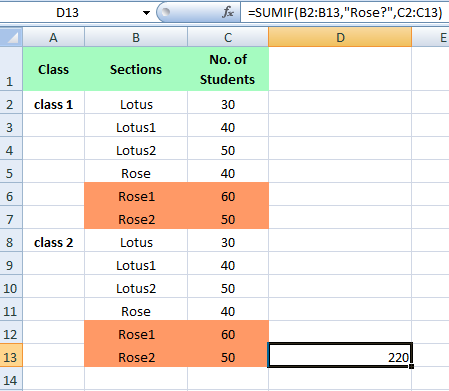>> The SUMIF function below sums values in the range if the corresponding cells in the other range contains the either criteria Text.
For example, for sum values in the range C2:C13 if the corresponding cells in the range B2:B13 contain the text Rose or Lotus exactly .
D13 equals =SUMIF(B2:B13,"Lotus",C2:C13)+SUMIF(B2:B13,"Rose",C2:C13)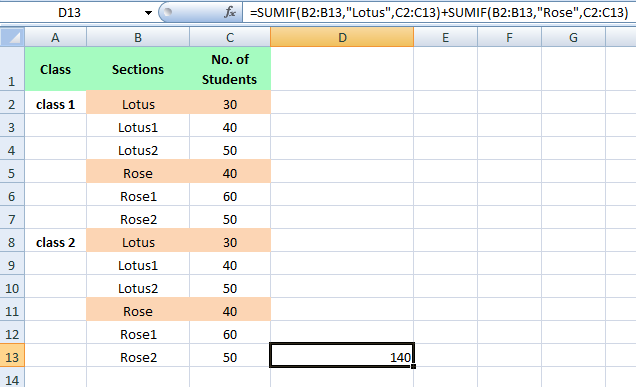### How to Sum Dates using SUMIF Function

>> The SUMIF function below sums the values in the range after the selected criteria Date
For example, for sum the Number of admissions after 13 july 2018,
D12 equals =SUMIF(B2:B11,">"&DATE(2018,7,13),D2:D11)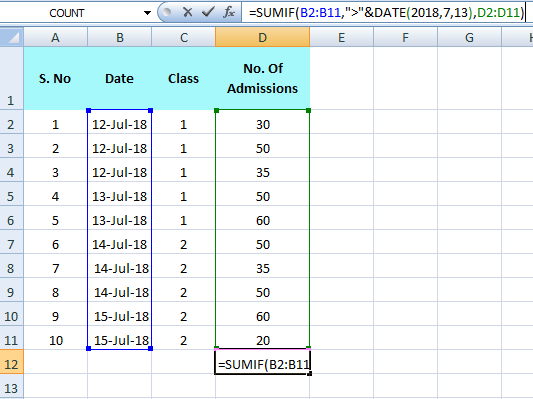DATE function in Excel accepts three arguments: year, month and day.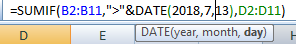Likewise , Any value can be Added According to dates.